# Laurent series

Jump to: navigation, search

A generalization of a power series in non-negative integral powers of the difference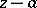or in non-positive integral powers ofin the form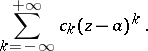(1)

The series (1) is understood as the sum of two series: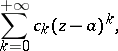the regular part of the Laurent series, andthe principal part of the Laurent series. The series (1) is assumed to converge if and only if its regular and principal parts converge. Properties of Laurent series: 1) if the domain of convergence of a Laurent series contains interior points, then this domain is a circular annulus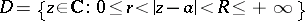with centre at the point; 2) at all interior points of the annulus of convergencethe series (1) converges absolutely; 3) as for power series, the behaviour of a Laurent series at points on the bounding circles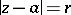and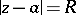can be very diverse; 4) on any compact set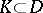the series (1) converges uniformly; 5) the sum of the series (1) inis an analytic function; 6) the series (1) can be differentiated and integrated interm-by-term; 7) the coefficients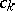of a Laurent series are defined in terms of its sumby the formulas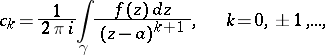(2)

where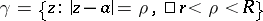is any circle with centresituated in; and 8) expansion in a Laurent series is unique, that is, if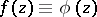in, then all the coefficients of their Laurent series in powers ofcoincide.

For the case of a centre at the point at infinity,, the Laurent series takes the form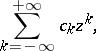(3)

and now the regular part iswhile the principal part is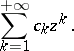The domain of convergence of (3) has the form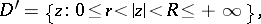and formulas (2) go into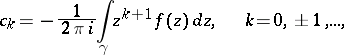where. Otherwise all the properties are the same as in the case of a finite centre.

The application of Laurent series is based mainly on Laurent's theorem (1843): Any single-valued analytic functionin an annuluscan be represented inby a convergent Laurent series (1). In particular, in a punctured neighbourhoodof an isolated singular pointof single-valued character an analytic functioncan be represented by a Laurent series, which serves as the main instrument for investigating its behaviour in a neighbourhood of an isolated singular point.

For holomorphic functionsof several complex variables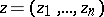the following proposition can be regarded as the analogue of Laurent's theorem: Any function, holomorphic in the productof annuli, can be represented inas a convergent multiple Laurent series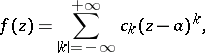(4)

is which the summation extends over all integral multi-indices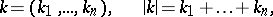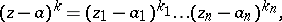whereis the product of the circles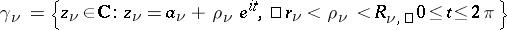,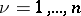. The domain of convergence of the series (4) is logarithmically convex and is a relatively-complete Reinhardt domain. However, the use of multiple Laurent series (4) is limited, since forholomorphic functionscannot have isolated singularities.